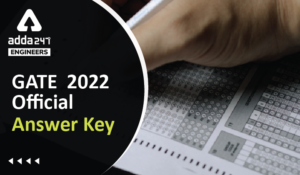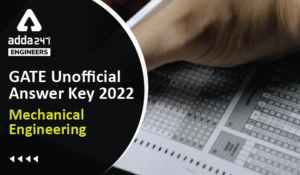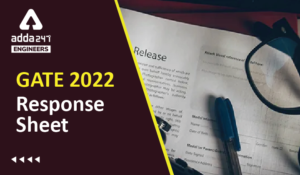Engineering Jobs   »   Civil Engineering Quiz

# GATE’22 CE : Weekly Practices Quiz: 22.08.2021

Quiz: Civil Engineering
Exam: GATE
Topic: Miscellaneous

Each question carries 2 marks
Negative marking: 1/3 mark
Time: 20 Minutes

Q1. A mild steel bar of square cross-section 40 mm × 40 mm is 400 mm long it is subjected to a longitudinal tensile stress of 440 N/mm² and lateral compressive stress is 200 N/mm² in perpendicular directions. E = 2 × 10^5 N/mm², μ = 0.3. what is the approximate elongation of the bar in the longitudinal direction?
(a) 0.44 mm
(b) 0.88 mm
(c) 0.22 mm
(d) 1 mm

Q2. For the plane frame as shown in the figureThe degree of kinematic indeterminacy, neglecting axial deformation, is
(a) 3
(b) 5
(c) 7
(d) 9

Q3. An embankment is to be constructed with a granular soil (bulk unit weight =20 kN/m^3) on a saturated clayey silt deposit (undrained shear strength = 25 kPa). Assuming undrained general shear failure and bearing capacity factor of 5.7. the maximum height (in m) of the embankment at the point of failure is
(a) 7.1
(b) 5.0
(c) 4.5
(d) 2.5

Q4. The distance between the points measured along a slope is 428 m. if the difference in level is 62 m, then the horizontal distance between them is
(a) 643.48 m
(b) 423.48 m
(c) 523.48 m
(d) 323. 48 m

Q5. The BOD_5 of a surface water sample is 200 mg/litre at 20°C. the value of the reaction constant is K = 0.2 day^(-1)with base ‘e’. the ultimate BOD of the sample is
(a) 126 mg/litre
(b) 544 mg/litre
(c) 146 mg/litre
(d) 316 mg/litre

Q6. A layer of normally consolidated, saturated silty clay of 1m thickness is subjected to one dimensional consolidation under a pressure increment of 20 kPa. The properties of the soil are: specific gravity = 2.7, natural moisture content = 45% compression index = 0.45% and recompression index = 0.05. the initial average effective stress within the layer is 100 kPa. Assuming Terzaghi’s theory to be applicable, the primary consolidation settlement (rounded off to the nearest mm) is
(a) 2 mm
(b) 9 mm
(c) 14 mm
(d) 16 mm

Q7. A road is having a horizontal curve of 400 m radius on which a super-elevation of 0.07 is provided. The coefficient of lateral friction mobilized on the curve when a vehicle is travelling at 100 kmph is
(a) 0.07
(b) 0.13
(c) 0.15
(d) 0.4

Q8. Probability of a 10 years flood to occur at least once in 4 years is ………….
(a) 50%
(b) 35%
(c) 25%
(d) 65%

Q9. The dynamic viscosity of a liquid is 1.2 × 10^(-4) N-s/m³, whereas, the density is 500 kg/m³. the kinematics viscosity in m²/s is
(a) 2.4×10^(-8) m²\/s
(b) 12×10^8 m²\/s
(c) 14×10^(-8) m²\/s
(d) 20×10^(-8) m²\/s

Q10. If the shrinkage factor of a map is 24/25 and R.F. is 1/2400, then correct R.F. for map will be
(a) 1/600
(b) 1/2500
(c) 1/2400
(d) 1/60000

Solutions

S1. Ans.(d)
Sol. Given,
A = 40×40 mm^2
L=400 mm
σ_x=400 N\/mm^2
σ_y = -200 N\/mm^2
E=2×10^5 N\/mm^2
μ=0.3
∆L= ?
Strain in longitudinal direction (∈_x ) will be-
∈_x=σ_x/E-μ σ_y/E
∆L/L=1/E (σ_x-μ σ_y )
∆L/400= 1/(2×10^5 ) [(400-0.3×(-200)]
▭(∆L=1 mm.)

S2. Ans.(b)
Sol. No. of joints (j) = 4
No. of member (m) = 3
No. of external reaction (re) = 5
Degree of kinematic indeterminacy (D_k) = ?
D_k=3j-re-m-r_r where,(r_r=Addition DOF due to joint hinge)
=(3×4)-5-3+1
=12-7
▭(D_k=5)

S3. Ans.(a)
Sol. Given, y_b=20kN\/m^3
Shear strength =S=C=25kN\/m^2
N_c=5.7
D_f=?
q_u=CN_C
y D_f=25×5.7 (∵q_u=yD_f )
20×D_f=25×5.7
▭(D_f=7.125m.)

S4. Ans.(b)
Sol.AB=62 m.
BC=428 m.
AC= ?
From Pythagoras theorem
BC^2=AB^2+AC^2
AC^2=(428)^2-(62)^2
AC= √((428)^2-(62)^2 )
= √((428+62)(428-62) )
=√(490×366)
▭(AC=423.48 m)

S5. Ans.(d)
Sol. Given,
BOD_5=200 mg\/l.
K=0.2\/day (at base e)
=0.2×0.434 (at base 10)
=0.0868
Ultimate BOD (L)= ?
▭(BOD_5=L(1-10^(-K_D t) ) )
200=L(1-10^(-0.0868×5) )
L=200/((1-10^(-0.0868×5) ) )
▭(L=316.52 mg\/l)

S6. Ans.(d)
Sol. Given,
H_0=1m.
∆σ ̅=20 kPa
G=2.7
w=0.45
C_C =0.45
(σ_0 ) ̅=100 kPa
∆H= ?
▭(∆H=(C_C H_O)/(1+e_0 ) log_10 [((σ_0 ) ̅+(∆σ) ̅)/(σ_0 ) ̅ ] )
We know-
Se=wG
1×e=0.45×2.7
▭(e=1.215)
∆H=(0.45×1)/(1+1.215) log_10 [(100+20)/100]
=0.45/2.215 log_10 (120/100)
=0.016 m
▭(∆H=16mm.)

S7. Ans.(b)
Sol. Given,
V=100 kmph
e=0.07
R=400m
f= ?
R=V^2/127(e+f)
400=(100)^2/127(0.07+f)
0.07+f=0.196
▭(f=0.13)

S8. Ans.(b)
Sol. T = 10 years, n = 4 years
P =1/T=1/10=0.1
q=1-P
q=1-0.1
q=0.9
Probability of a 10 years flood to occur at least once in 4 years = 1 – q^n
=1-(0.9)^4
=0.3439≃0.35=35%

S9. Ans.(a)
Sol. Given, Dynamic viscosity (μ) = 1.2×10^(-4) (N-s)/m^2
Density of fluid (ρ) = 500 kg/m³
= 5000 N/m³
Kinematic viscosity (γ) = μ/ρ
=(1.2×10^(-4))/5000
▭(γ=2.4×10^(-8) m^2 \/sec.)

S10. Ans.(b)
Sol. Shrinkage factor of old map =24/25
Incorrect scale (R.F) = 1/2400
Correct scale = shrinkage factor × incorrect scale
=24/25×1/2400
=1/2500

Sharing is caring!

Thank You, Your details have been submitted we will get back to you.
•HURL Recruitment 2022 Apply Online for 1...
•GATE 2022 Scorecard Released, Check Step...
•GATE Result 2022 OUT Live Check Your GAT...
•GATE 2022 Official Answer Key Out, Downl...
•GATE 2022 Answer Key Mechanical Engineer...
•GATE 2022 Response Sheet, Direct Link to...